NCERT Solutions for Class 8 Maths Chapter 13 Direct and Indirect Proportions Ex 13.1 are part of NCERT Solutions for Class 8 Maths. Here we have given NCERT Solutions for Class 8 Maths Chapter 13 Direct and Indirect Proportions Ex 13.1.

 Board CBSE Textbook NCERT Class Class 8 Subject Maths Chapter Chapter 13 Chapter Name Direct and Indirect Proportions Exercise Ex 13.1 Number of Questions Solved 10 Category NCERT Solutions

## NCERT Solutions for Class 8 Maths Chapter 13 Direct and Indirect Proportions Ex 13.1

Question 1.
Following are the car parking charges near a railway station up to
4 hours ₹ 60
8 hours ₹ 100
12 hours ₹ 140
24 hours ₹ 180
Check if the parking charges are in direct proportion to the parking time.
Solution.
We have
$$\frac { 60 }{ 4 } =\frac { 15 }{ 1 }$$
$$\frac { 100 }{ 8 } =\frac { 25 }{ 2 }$$
$$\frac { 140 }{ 12 } =\frac { 35 }{ 3 }$$
$$\frac { 180 }{ 24 } =\frac { 15 }{ 2 }$$
Since all the values are not the same, therefore, the parking charges are not in direct proportion to the parking time.

Question 2.
A mixture of paint is prepared by mixing 1 part of red pigments with 8 parts of the base. In the following table, find the parts othe f base that need to be added.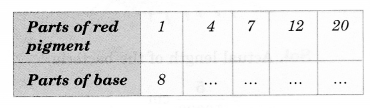Solution.
Sol. Let the number of parts of red pigment is x and the number of parts of the base is y.
As the number of parts of red pigment increases, a number of parts of the base also increases in the same ratio. So it is a case of direct proportion.
We make use of the relation of the type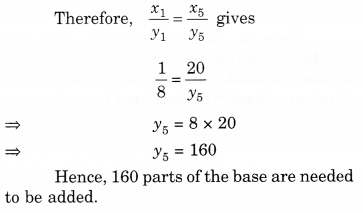Question 3.
In Question 2 above, if 1 part of a red pigment requires 75 mL of the base, how much red pigment should we mix with 1800 mL of base?
Solution.
Let the number of parts of red pigment is x and the amount of base be y mL.
As the number of parts of red pigment increases, the amount of base also increases in the same ratio. So it is a case of direct proportion. We make use of the relation of the type.Question 4.
A machine in a soft drink factory fills 840 bottles in six hours. How many bottles will it fill in five hours?
Solution.
Let the machine fill x bottles in five hours. We put the given information in the form of a table as shown below :
Number of bottles filled 840 x2
Number of hours 6 5
More the number of hours, more the number of bottles would be filled. So, the number of bottles filled and the number of hours are directly proportional to each other.
So, $$\frac { { x }_{ 1 } }{ { x }_{ 2 } } =\frac { { y }_{ 1 } }{ { y }_{ 2 } }$$
⇒ $$\frac { 840 }{ { x }_{ 2 } } =\frac { 6 }{ 5 }$$
⇒ $$6{ x }_{ 2 }=840\times 5$$
⇒ $${ x }_{ 2 }=\frac { 840\times 5 }{ 6 }$$
⇒ $${ x }_{ 2 }=700$$
Hence, 700 bottles will be filled.

Question 5.
A photograph of a bacteria enlarged 50,000 times attains a length of 5 cm as shown in the diagram. What is the actual length of the bacteria ? If the photograph is enlarged 20,000 times only, what would be its enlarged length?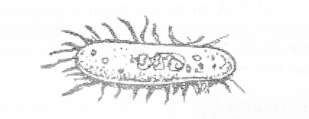Solution.
Actual length of the bacteria
= $$\frac { 5 }{ 50000 }$$cm
= $$\frac { 1 }{ 10000 }$$ = $${ 10 }^{ -4 }$$cm
10000
Let the enlarged length be y2 cm. We put the given information in the form of a table as shown below:
Number of times Length attained
photograph enlarged (in cm)
50.000 5
20.000 y2
More the number of times a photograph of a bacteria is enlarged, more the length attained. So, the number of times a photograph of a bacteria is enlarged and the length attained are directly proportional to each other.
So,$$\frac { { x }_{ 2 } }{ { y }_{ 2 } } =\frac { { x }_{ 2 } }{ { y }_{ 2 } }$$
⇒ $$\frac { 50000 }{ 5 } =\frac { 20000 }{ { y }_{ 2 } }$$
⇒ $$50000{ y }_{ 2 }=5\times 20000$$
⇒ $${ y }_{ 2 }=\frac { 5\times 20000 }{ 50000 }$$
⇒ $${ y }_{ 2 }=2$$
Hence, its enlarged length would be
2 cm.

Question 6.
In a model of a ship, the mast is 9 cm high, while the mast of the actual ship is 12 m high. If the length of the ship is 28 m, how long is the model ship ?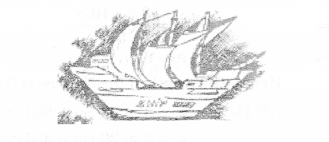Solution.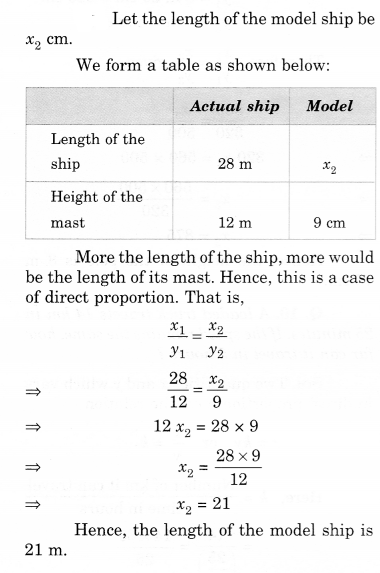Question 7.
Suppose 2 kg of sugar contains 9 x 106 crystals. How many sugar crystals are there in
(i) 5 kg of sugar?
(ii) 1.2 kg of sugar?
Solution.
Suppose the amount of sugar is x kg and the number of crystals is y.
We put the given information in the form of a table as shown below:As the amount of sugar increases, the number of crystals also increases in the same ratio. So it is a case of direct proportion. We make use of the relation of the type $$\frac { { x }_{ 1 } }{ { y }_{ 1 } } =\frac { { x }_{ 2 } }{ { y }_{ 2 } }$$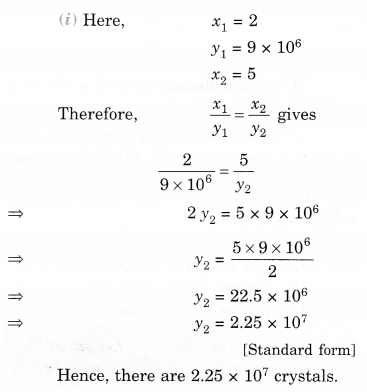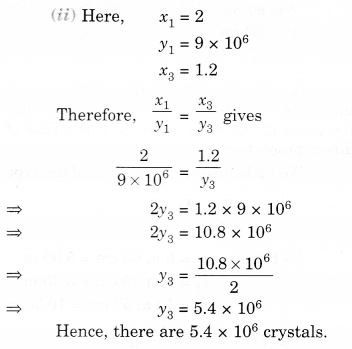Question 8.
Rashmi has a roadmap with a scale of 1 cm representing 18 km. She drives on a T’oad for 72 km. What would be her distance covered in the map?
Solution.
Let the distance covered in the map be x cm. Then,
1 : 18 = x : 72
⇒ $$\frac { 1 }{ 18 } =\frac { x }{ 72 }$$
⇒ $$x=\frac { 72 }{ 18 }$$
⇒ x = 4
Hence, the distance covered in the map would be 4 cm.

Question 9.
A5 m 60 cm high vertical pole casts a shadow 3 m 20 cm long. Find at the same time
(i) the length of the shadow cast by another pole 10 m 50 cm high
(ii) the height of a pole which casts a shadow 5 m long.
Solution.
Let the height of the vertical pole be x m and the length of the shadow be y m.
We put the given information in the form of a table as shown below: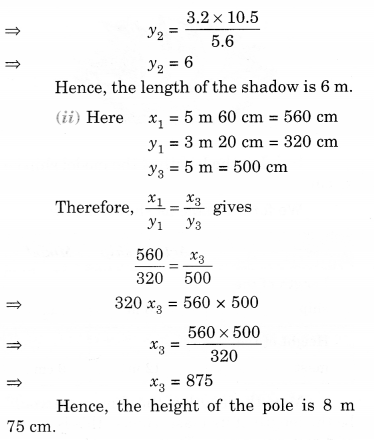Question 10.
A loaded truck travels 14 km. in 25 minutes. If the speed remains the same, how far can it travel in 5 hours?
Solution.
Two quantities x and y which vary in direct proportion have the relation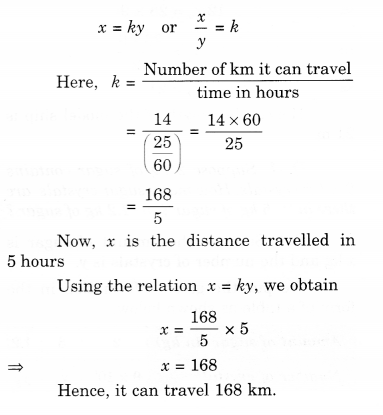We hope the NCERT Solutions for Class 8 Maths Chapter 13 Direct and Indirect Proportions Ex 13.1 help you. If you have any query regarding NCERT Solutions for Class 8 Maths Chapter 13 Direct and Indirect Proportions Ex 13.1, drop a comment below and we will get back to you at the earliest.